## Given problem

There is an integer array `nums` sorted in ascending order (with distinct values).

Prior to being passed to your function, `nums` is possibly rotated at an unknown pivot index k (`1 <= k < nums.length`) such that the resulting array is `[nums[k], nums[k+1], ..., nums[n-1], nums, nums, ..., nums[k-1]]` (0-indexed).

For example, `[0,1,2,4,5,6,7]` might be rotated at pivot index 3 and become `[4,5,6,7,0,1,2]`.

Given the array nums after the possible rotation and an integer target, return the index of `target` if it is in `nums`, or `-1` if it is not in `nums`.

You must write an algorithm with `O(log n)` runtime complexity.

Example 1:

• Input: nums = `[4,5,6,7,0,1,2]`, target = 0
• Output: 4

Example 2:

• Input: nums = `[4,5,6,7,0,1,2]`, target = 3
• Output: -1

Example 3:

• Input: nums = ``, target = 0
• Output: -1

Constraints:

• `1 <= nums.length <= 5000`
• `-104 <= nums[i] <= 104`
• All values of `nums` are unique.
• `nums` is an ascending array that is possibly rotated.
• `-104 <= target <= 104`

## Using Linear Search to find element

Normally, the first thing we do is to use brute-force to deal with problem. In this case, we will use linear search to do it.

``````public static int search(int[] nums, int target) {
int pos = 0;
for (int i = 0; i < nums.length - 1; ++i) {
if (nums[i] == target) {
pos = i;
break;
}
}

return pos;
}
``````

The complexity of using Linear Search is:

• Time complexity: O(n)
• Space complexity: O(1)

The drawback of using Linear Search is about the time complexity - O(n). But we can improve it in O(logn), because our rotated array has sub-arrays that are increased array.

So we can use Binary Search.

• First way.

Next, we will analyze our problem when using Binary Search’s the first invariant.

1. Case 1 - When we jump into the right sub-arrayBut in this case, we can have two issues:

• our target will under this range.

For example: target = 2, then we have: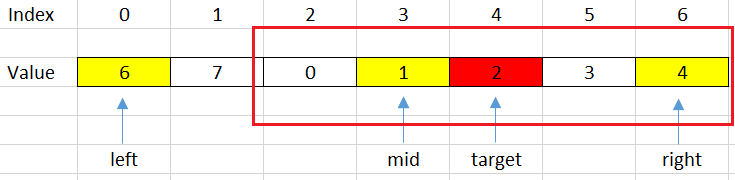Then, we will shift left with steps: `left = mid + 1;`

• our target will not be contain in this range.

For example: target = 7, then we have: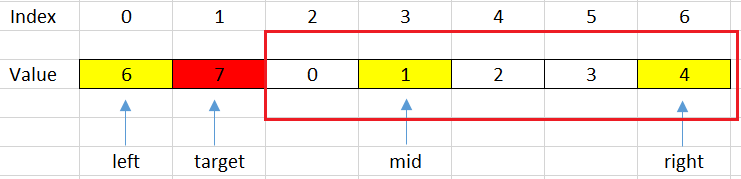Then, we will shift right with steps: `right = mid - 1;`

Based on the above conditions, we can have the segment code:

`````` if (nums[mid] <= nums[right]) {
if (nums[mid] < target && target <= nums[right]) {
left = mid + 1;
} else {
right = mid - 1;
}
}
``````
2. Case 2 - When we jump into the left sub-array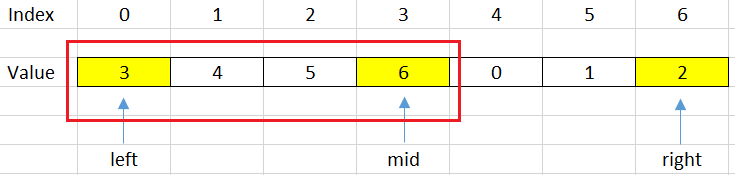But in this case, we also have two issues:

• our target will under this range.

For example: target = 4, it can be described in a below image.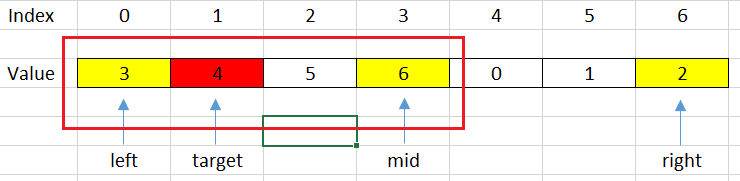Then, we will shift left with some steps: `right = mid - 1;`

• our target will be not contain in this range.

For example, target = 1, then we have: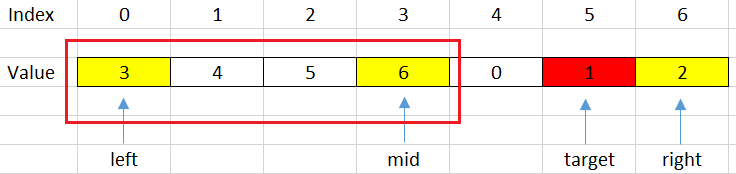Then, we will shift right with some steps: `left = mid + 1;`

Based on above conditions, we can some code to describe it.

`````` if (nums[mid] >= nums[left]) {
if (nums[left] < target && target < nums[mid]) {
right = mid - 1;
} else {
left = mid + 1;
}
}
``````

Below is our source code of this way:

• First invariant of Binary Search.

``````  public static int search(int[] nums, int target) {
int left = 0;
int right = nums.length - 1;

while (left <= right) {
int mid = left + (right - left) / 2;
if (nums[mid] == target) {
return mid;
}

if (nums[mid] <= nums[right]) { // right half is sorted
if (target > nums[mid] && target <= nums[right]) {
left = mid + 1;
} else {
right = mid - 1;
}
} else {        // left half is sorted
if (nums[left] <= target && target < nums[mid]) {
right = mid - 1;
} else {
left = mid + 1;
}
}
}

return -1;
}
``````
• Third invariant of Binary Search.

``````  class Solution {
public int search(int[] nums, int target) {
int left = 0;

// The initially condition of right variable is not correct when using the template of Binary Search's third invariant.
// But in this problem, we need to use it to pass all test case in LeetCode.
int right = nums.length - 1;

while (left + 1 < right) {
int mid = left + (right - left) / 2;

if (nums[mid] == target) {
return mid;
} else if (nums[mid] > nums[left]) {    // 1st sorted sub-array
if (nums[left] <= target && target <= nums[mid]) {
right = mid;
} else {
left = mid;
}
} else {    // 2nd sorted sub-array
if (right < nums.length && nums[mid] <= target && target <= nums[right]) {
left = mid;
} else {
right = mid;
}
}
}

if (nums[left] == target) {
return left;
}

if (right < nums.length && nums[right] == target) {
return right;
}

return -1;
}
}
``````
• Second way.

We can search pivot point in this sorted rotated array. Then, search the `target` on each sub-array.

``````  class Solution {
public int search(int[] nums, int target) {
int pivot = findPivot(nums);

int res = binarySearch(nums, 0, pivot, target);
if (res != -1) {
return res;
}

return binarySearch(nums, pivot, nums.length, target);
}

private int findPivot(int[] nums) {
int left = 0;
int right = nums.length;

while (left + 1 < right) {
int mid = left + (right - left) / 2;

if (nums[mid - 1] > nums[mid]) {
return mid;
} else if (nums[mid] > nums[left]) {
left = mid;
} else {
right = mid;
}
}

return nums[left] > nums ? 0 : left;
}

private int binarySearch(int[] nums, int startIdx, int endIdx, int target) {
int left = startIdx;
int right = endIdx;

while (left + 1 < right) {
int mid = left + (right - left) / 2;

if (nums[mid] == target) {
return mid;
} else if (nums[mid] < target) {
left = mid;
} else {
right = mid;
}
}

if (nums[left] == target) {
return left;
}

if (right < nums.length - 1 && nums[right] == target) {
return right;
}

return -1;
}
}
``````

The complexity of Binary Search is:

• Time complexity: O(log(n)).
• Space complexity: O(1).

## Wrapping up

• Understanding why we can apply Binary Search in this problem.

• Go from the brute-force solution, then optimize it.